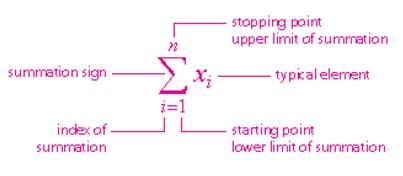# SUMMATION FORMULA

When large number of data are concerned, then summation is needed quite often. To write a very large number, summation notation is useful.  The sequence [1,2,4,2..] whose value is the sum of the each number in the sequence is summation. In simple words, summation notation helps write a short form for addition of very large number of data. We use this symbol –

We use this symbol –  , called sigma to denote summation. When a sequence is needed to add from left to right, it could run intermediate result in a partial sum, running total or prefix sum.

The form in which the summation notation is used:

$$\begin{array}{l}\sum_{i=n}^{m}a_{i}=a_{n}+a_{n+1}+a_{n+2}+….+a_{m-2}+a_{m-1}+a_{m}\end{array}$$

To make it clear, read what each notation in the summation formula stands for:This expression is read as “The sum of x sub i from i equals 1 to n”.

### Solved Example

Question: Evaluate:

$$\begin{array}{l}\sum_{x-0}^{4}x^{4}\end{array}$$

Solution:

$$\begin{array}{l}\sum _{k=0}^n\:=\:a_0\:+\:\sum _{k=1}^n\end{array}$$

$$\begin{array}{l}a_0=0^4=0\end{array}$$

$$\begin{array}{l}=0+\sum _{n=1}^4n^4\end{array}$$

$$\begin{array}{l}\sum _{n=1}^4n^4\end{array}$$

$$\begin{array}{l}\mathrm{Apply\:the\:Sum\:Formula:\quad }\sum _{k=1}^nk^4=\frac{1}{30}n\left(n+1\right)\left(2n+1\right)\left(3n^2+3n-1\right)\end{array}$$

$$\begin{array}{l}=\frac{1}{30}\cdot \:4\left(4+1\right)\left(2\cdot \:4+1\right)\left(3\cdot \:4^2+3\cdot \:4-1\right)\end{array}$$

$$\begin{array}{l}=354\end{array}$$

## Video Lesson

### Formulas for Summation